Home » Voltage Converter » How to Make a Step-up 110 volt to 220 volt Voltage Converter?

# How to Make a Step-up 110 volt to 220 volt Voltage Converter?

For 110 volt to 220 volt voltage converter, how to calculate the copper wire turns of voltage converter primary and secondary winding, how to choose the size of silicon steel sheet, and how to calculate the diameter of copper wire?

For reference:
Simple calculation of voltage converters:
1. The number of turns per volt
Number of turns per volt=55/core section
For example, your core section=3.5X1.6=5.6cm^2, so the number of turns per volt=55/5.6=9.8turns
2. The number of coil turns
Primary coil n1=220X9.8=2156turns
Secondary coil n2=110X9.8X1.05=1617turns
1.05 in the calculation of the secondary coil turns is to the pressure drop considering load.
3. The wire diameter
Assume the output voltage is 110V and the output current is 2A.
Voltage converter output capacity=110X2=220VA
Input capacity=voltage converter output capacity/0.8=275VA
Primary coil current I1=275/220=1.25A
Wire diameter d=0.8√I
Primary coil wire diameter d1=0.8√I1=0.8√1.25=0.9mm
Secondary coil wire diameter d2=0.8√I2=0.8√2=1.14mm
Assume that the input of the power converter is connected to 220 volts. Then:
1. Turns ratio: Primary voltage/secondary voltageX1.05, which is 220V/secondary voltageX1.05.
2. Determination of the number of primary circles: 40 to 50 divided by the core cross-sectional area (empirical formula), depending on the quality of the core is good or poor, good core can take 40, poorer core can take 50.
3. Core cross-sectional area: S=1.2X√(Power/Efficiency)
4. Copper cross-sectional area: according to the current calculation, the general take 2.5A per square millimeter. The primary current of the voltage converter is the power/220V; the secondary current is the power/secondary voltage.
Step-up voltage converter working principle: Step-up voltage converter is to transform low AC voltage, high current, low impedance to high AC voltage, low current and high impedance devices. When the primary coil through the AC current, the iron core (or magnetic core) will produce AC magnetic flux, the secondary coil induces a voltage (or current). The input voltage must be alternating current source whose output voltage is proportional to the turns’ ratio of the output input coil.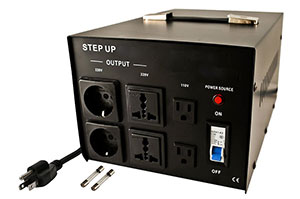Formula and description: The number of turns in the two sets of voltage converter respectively is N1 and N2, N1 is the primary and N2 is secondary. An alternating voltage is applied to the primary winding to induce electromotive force at both ends of the secondary coil. When N2>N1, The induced electromotive force is even higher than the voltage applied to primary. This is called the step-up voltage converter. The primary secondary voltage and the number of coils has the following relationship:
N=U1/U2=N1/N2, where n is called the voltage ratio (turns ratio). When n>1, then N1<N2, U1<U2, the voltage converter is a step-up voltage converter. In addition, the current ratio I1/I2=N2/N1 and electric power P1=P2. Note that the above equation is valid only when the ideal voltage converter has only one secondary coil. P1=P2+P3 and U1/N1=U2/N2=U3/N3 when there are two secondary coils. Seeking the current must be calculated using the electric power relationship, when there are a number of categories.

Known core area is 3.5X3.2cm^2, the primary voltage 110V and secondary voltage 220V, seeking: Second turn and diameter, current. Silicon steel sheet is the voltage converter core cross-sectional area. Basically the other parameters of the voltage converter can be determined after the calculation. Finally, see whether your core window can put down the coil.
1. Know the core cross-sectional area, each turn of the voltage et=BXS/450, et is the voltage for each turn, B is the magnetic density. If it is ordinary voltage converter silicon steel sheet, dry-type voltage converter, can take 15-16.5 kilogauss (First calculated with 16). S is the area=3.5X3.2cm^2, would have multiplied by the laminated coefficient of 0.96, ignored here. 450 is a constant when operating frequency is 50Hz, et=16X3.5X3.2/450=0.398V/turn.
2. Seek the number of high and low voltage turns: the primary N1=110/0.398=276.39turns, take an integer of 277 turns; secondary N2=220/0.398=552.77turns, take an integer 553 turns.
3. First give the output power of the voltage converter, such as 50W. Then the output current I2=50/220=0.23A, primary current I1=50/110=0.46A.
4. The current density of this voltage converter can take about 2.5A?mm^2 or so. The cross-sectional area of the secondary line is 0.23/2.5=0.08mm^2
5. Check the line gauge (ordinary enameled wire-circular cross-section), secondary diameter is 0.33mm, the primary diameter is 0.51mm.
6. Then, the primary and secondary coils can be arranged. See the window area is whether enough, if not, reduce the power. If there is surplus, you can increase the power of the voltage converter.
Other instructions:
1. As for the temperature rise calculation, as long as the control of electromagnetic density, temperature rise is generally not very high, estimated at 60-70℃.
2. In order to make the voltage converter easy to use, you can increase 3%-5% of the primary turns, or reduce 1-2 turns of the primary turns.
The production method of step-up voltage converter
Make a power supply from 110V to 220V 2000W voltage converter. According to the power and core calculation formula:
S＝1.2X√P＝1.2X√2000≈54cm^2
Selected core cross-sectional area 40cm^2 is too small, it is possible to accommodate no less than winding. The calculation steps According to core cross-sectional area 54cm^2:
When core permeability is 10,000 Gauss,
the number of turns per volt: N=45/S=45/54≈0.84turns
Primary turns: 110X0.84≈92turns
Secondary turns (iron loss and copper loss impact of voltage converter shall be increased by 5% margin): 220×0.84×1.05≈194turns
Primary and secondary current:
I1＝P/U＝2000/110≈18.2A
I2＝2000/220≈9.1A
Primary and secondary wire diameter:
D＝1.13×√(I/δ)(Current density is 2.5-3A per square, take 2.5A calculation)
D1＝1.13×√18.2/2.5=3.05mm

Plz Calculate (2 * 6) =
(Your comment will show after approved.)

You may also like: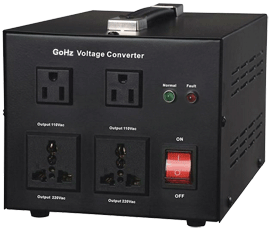With a Voltage Converter, you can convert
110v to 220v;
120v to 220v;
220v to 110v;
230v to 110v;
240v to 110v.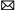info@VoltConverter.com
What Happens When an Appliance is ...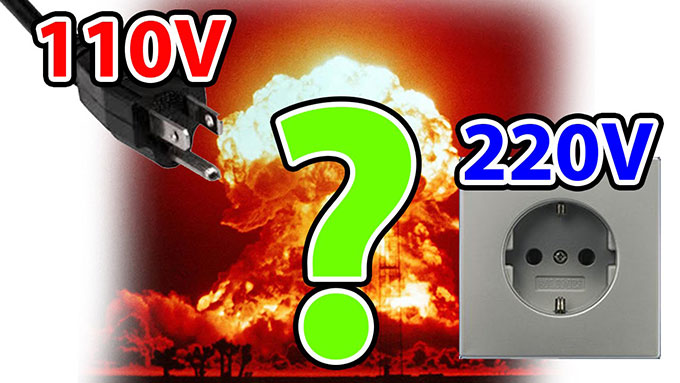If the 110V appliance is connected to a 220V power supply, the power may quadruple at the moment the appliance switched on, and ...
Automatic 220v to 110v Voltage ...As we know, electric voltage of some countries and regions is 220V. But in America, the electric voltage is generally 110V. When ...
Voltage Difference between US and UK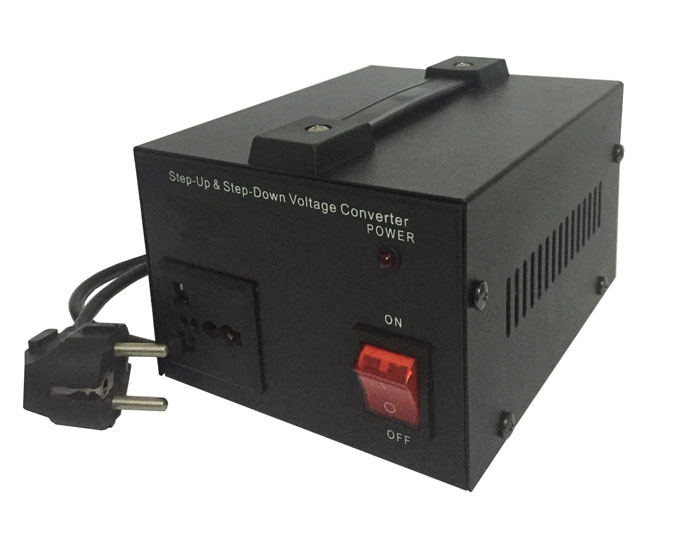Many people who travel to the United States found it difficult to use their electronic devices, because of different power supply ...